## Area Conversion Calculator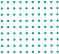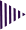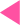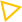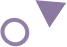=
Best Area conversion calculator

# Online area conversion calculator

Area calculator help you convert one measure of an area into another. This land unit converter can help you convert between many different units of measurement, including square meters, feet, hectares, area and square inches, square yard. square kilometer
You don't have to worry about that anymore with the area conversion calculator. Total calculators provide this tool is entirely online free, simple to use and easy to understand. You simply need to enter the value for the area you wish to convert, select the measurement unit, and then choose the unit you wish to convert it to.

### How do you calculate area conversion?

You can use the formula for the area of a square, A=s2 , to convert square units. Since 1 Meter = 3.28084 Foot, substitute s by 3.28 . So, 1 square Meter is equal to 10.763 square feet. Conversion rates are an effective way of comparing and contrasting the performance of multiple advertising channels. A conversion is defined as an exchange from one unit of measure to another, by multiplying or dividing.

### Area Conversion Units Definitions

• Acres (ac) converter definition:-

The acre is a unit of area in the imperial and U.S. customary systems. One acre equals 4046.873sq. Meter, 43560.178 Sq. Foot. or 0.405 hectare. 640 acres make 1 mile. The acre is often used in measurements for areas of land and its international symbol is ac. Acre is basicly used in United States and United Kingdom. It is also used in India and thouse countries under former British Colonial rule.

• Ares (a) converter definition:-

In the metric system,100 square meters is equal to one Ares (a). This is equal to 0.0247 acres. The hectare (equivalent to 100 ares) is the primary unit of land measurement for most nations.
Ares to other Measurement Conversions
100 ares :- 2.471 acres
100 ares :- 1 Hectare
100 ares :- 107639.104 Sq. Foot
100 ares :- 10000 Sq. Meeter
100 ares :- 0.01 Sq. Kilometer

• Hectare (ha) converter definition:-

- Hectare (ha), is a measurement of an area of land which is 1 hectare equal to 10,000 square metres, or 2.471 acres. A large part of land is primarily measured by this method throughout the world. The hectare is an accepted unit of measurement in the international system of units. Among the geographies, land measurement is most commonly used in Australia, Burma, Canada, the European Union, India, the US, and the UK.

• Square foot converter definition:-

A square foot is the standard size measurement for calculating an area. The square foot is the imperial unit of area and the U.S. customary unit of area. It is primarily used in the United States and partially in Canada, the United Kingdom, Bangladesh, India, Nepal, Pakistan, Ghana, Liberia, Malaysia, Myanmar, Singapore, and Hong Kong.
How do I calculate a room sq ft?
The length and width of each room are measured in feet. Multiply the length and width of a room to determine its square footage. An example: A bedroom measuring 14 feet by 20 feet is 280 square feet (14 x 20 = 280).

• Square meter converter definition:-

As a unit of measurement, square meters (sq m) are commonly denoted by m2.
We typically use a square meter to measure a two-dimensional space, such as a field or floor. The square meter is used to measure the area of a plot or land. For example, the area of a room, the area of the walls, the area of the floor and the area of the house first measured in feet and then be converted into square meters.